# AMSL and skiing

Tomas skis from point A (3200m above sea level to place B. Hill has 20% descent. The horizontal distance between the start and finish is 2,5km. At what altitude is point B?

Correct result:

B =  2700 m AMSL

#### Solution: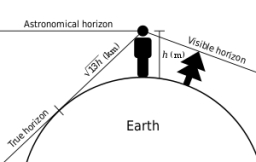We would be pleased if you find an error in the word problem, spelling mistakes, or inaccuracies and send it to us. Thank you!Tips to related online calculators
Do you want to convert length units?

## Next similar math problems:

• Current in the conductorCalculate the current in the conductor (in mA) if it is connected to a 4.5 V voltage source and its resistance is 20 (ohm).
• The bulbWhat is the resistance of a filament lamp when it is connected to a 9V battery and has a current of 120 mA?
• Seasonal saleThe bike became cheaper on the seasonal sale by 22%, which is 88 euros. At what price is it sold now?
• Clothes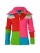CZK 2,400 cost clothes, a sweater by 150% than a T-shirt, trousers 2x more than a sweater, a jacket as a sweater and trousers together. What did the individual clothes cost?
• Two shopsIn two different shops, the same skis had the same price. In the first, however, they first became more expensive by 20% and then cheaper by 5%. In the second, they were first cheaper by 5% and then more expensive by 30%, so after these adjustments in the
• Tulips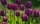Of the 120 tulips in the garden, 45 are red, 66 are yellow, the rest are brindle. What percentage of tulips in the garden are brindle?
• 14 yearsKája is 14 years old. Mom 44. How many years will mom be 4 times older?
• Proportional relationship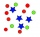The ordered pairs (6,24) and (1, s) represent a proportional relationship. Find the value of s.
• Pagans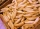Jano and Michael ate pagans. Jano ate three more than Michael. The product of their counts (numbers) is 180. How many pagans did each of them eat?
• Trapezoid 25Trapezoid PART with AR||PT has (angle P=x) and (angle A=2x) . In addition, PA = AR = RT = s. Find the length of the median of Trapezoid PART in terms of s.
• One dice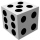Calculate the probability of a roll of one dice with the numbers 1, 2, 3, 4, 5, 6 on the walls. Write the results in a notebook in the shape of a fraction in the basic form: 2/3. a, The number 1 falls on the cube. b, The number 5 falls on the cube. c, An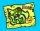There are 4 roads from city A to city B. There are 5 roads from city B to city C. How many different routes can we come from city A to city C via city B?Kamila wrote all natural numbers from 1 to 400 inclusive. How many times did she write the number 4?The staircase has a total height of 3.6 m and forms an angle of 26° with the horizontal. Calculate the length of the whole staircase.A sphere segment is cut off from a sphere k with radius r = 1. The volume of the sphere inscribed in this segment is equal to 1/6 of the volume of the segment. What is the distance of the cutting plane from the center of the sphere?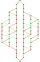Write all two-element combinations from elements a, b, c, d.How long is a ladder that touches on a wall 4 meters high and its lower part is 3 meters away from the wall?